# C Decision Making

C supports the following two types of decision making statements:

You can further divide both the above statements into the following parts:

## C Decision Making Statements Example

Here is an example demonstrates how to use decision making statements in C programming to control the program.

```/* C Decision Making Example */

#include<stdio.h>
#include<conio.h>
void main()
{
int num;
clrscr();
printf("Enter a number: ");
scanf("%d", &num);
if(num>10)
{
printf("The number is greater than 10.");
}
else
{
printf("The number is less than 10.");
}
getch();
}```

Here are the two sample runs of the above C decision making program: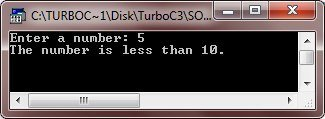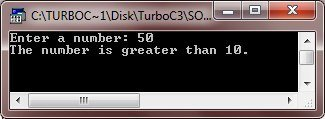Let's take another program on decision making or selection statements in C.

```/* C Decision Making Program */

#include<stdio.h>
#include<conio.h>
void main()
{
int num;
clrscr();
printf("Enter 10.");
scanf("%d", &num);
if(num == 10)
printf("Well Done!");
else
printf("Oops!");
getch();
}```

Here are the two sample runs of the above selection statements program in C.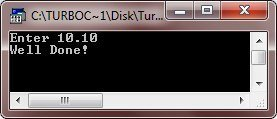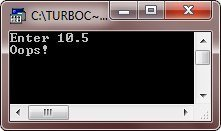Here is another example also demonstrates decision making statement in C

```/* C Decision Making */

#include<stdio.h>
#include<conio.h>
void main()
{
int mark;
clrscr();
printf("Enter marks obtained: ");
scanf("%d", &mark);
if(mark>80)
printf("You are passed!");
else
printf("You are failed!");
getch();
}```

Here are the two sample runs of the above C decision making program: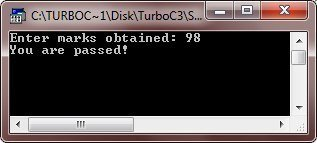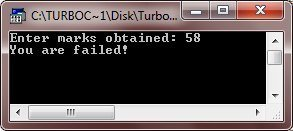### More Examples

Here are the list of some more examples that you can go for:

Tools
Calculator